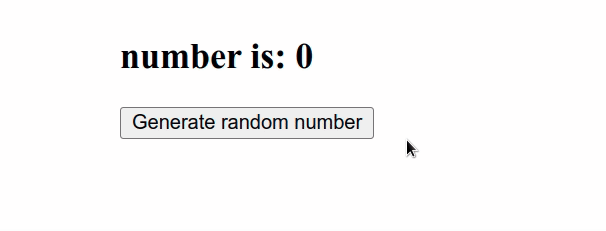# Generate a random number in ReactWed Apr 20 20222 min read## Generate a random number in React#

Use the `Math.random()` function to generate a random number in React, e.g. `Math.floor(Math.random() * (max - min + 1)) + min`. The `Math.random` function returns a number in the range 0 to less than 1 but can also be used to generate a number in a specific range.

App.js
```Copied!```import {useState} from 'react';

const App = () => {
const [num, setNum] = useState(0);

function randomNumberInRange(min, max) {
// 👇️ get number between min (inclusive) and max (inclusive)
return Math.floor(Math.random() * (max - min + 1)) + min;
}

const handleClick = () => {
setNum(randomNumberInRange(1, 5));
};

return (
<div>
<h2>number is: {num}</h2>
<button onClick={handleClick}>Generate random number</button>
</div>
);
};

export default App;
``````

The code snippet above uses the Math.random() function to generate a number in the range `1` (inclusive) to `5` (inclusive).If you don't need to generate a number in a specific range, but simply need a random number, you can use the `Math.random()` function directly.

App.js
```Copied!```console.log(Math.random()); // 👉️ 0.9349907593750104
console.log(Math.random()); // 👉️ 0.1028502928025743
console.log(Math.random()); // 👉️ 0.9268013352071363
``````

If you need to generate a random number every N seconds and render it in your component, use the `useEffect` hook to set up the interval.

App.js
```Copied!```import {useEffect, useState} from 'react';

const App = () => {
const [num, setNum] = useState(0);

function randomNumberInRange(min, max) {
// 👇️ get number between min (inclusive) and max (inclusive)
return Math.floor(Math.random() * (max - min + 1)) + min;
}

useEffect(() => {
const interval = setInterval(() => {
// 👇️ generate random number between 1 and 10
setNum(randomNumberInRange(1, 10));
}, 1000); // 👈️ runs every 1 second

return () => {
clearInterval(interval);
};
}, []);

return (
<div>
<h2>number is: {num}</h2>
</div>
);
};

export default App;
``````

The example above uses the setInterval() method to invoke a function every `1` second.

The second parameter we passed to the `setInterval()` method is the delay (in milliseconds) between invocations of the function.

If you don't pass an argument for the `delay`, it defaults to `0`.

In the function, we generate a random number between `1` and `10` and update the state variable.

The function we returned from the useEffect hook is ran when the component unmounts.

We used the clearInterval() method to cancel the repeating action that we set up using `setInterval`.

This is something you should always do when using methods like `setInterval()` or `addEventListener()`, because if you don't clean up when the component gets unmounted, you would get a memory leak in your application.

Use the search field on my Home Page to filter through my more than 1,000 articles.